Home > A2C > Chapter 7 > Lesson 7.1.5 > Problem7-78

7-78.
1. Rewrite each expression below as an exponential expression with a base of 2. Homework Help ✎

1. 16

2.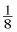3.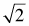4.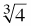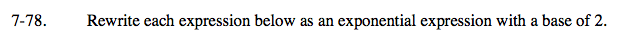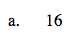2 to ___ power = 16?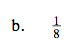$2^3 = 8 \text{ and } 2^{-1} = \frac{1}{2}$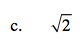Raise the base to a fractional power.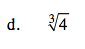$2^\small{ \frac{2}{3} }$